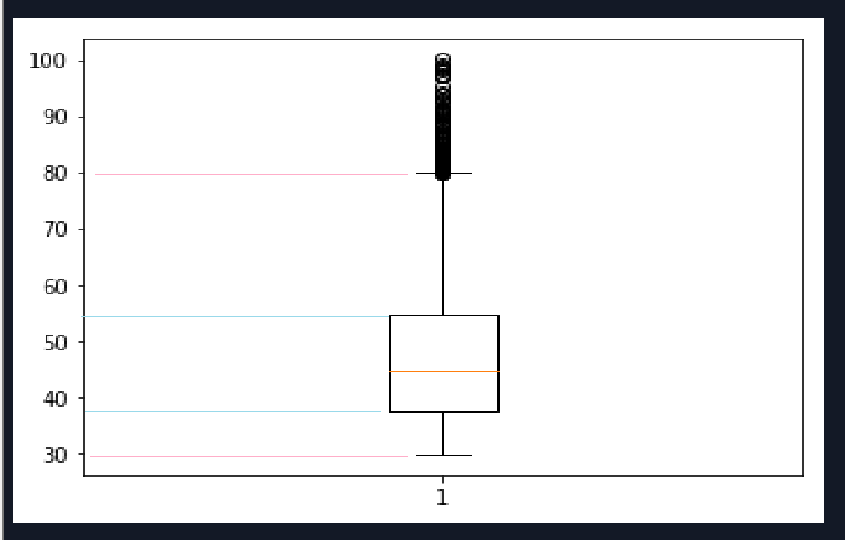# AdventureWorks DW Series 5 : Box PLot to identify Outliers and Targeted Cutomers in PythonPrerequisites To Follow this Exercise :

2. Python Installed in your System : If you are a new user to Python and want to know how to install Python via the Anaconda Distribution , You can go through the step by step Blog i have written to install Python via Anaconda Distribution & start using Jupyter Notebook : https://storejockey.com/2019/06/09/python-anaconda-distribution-how-to-download-and-install-it-and-run-the-first-python-program/

Once you have the Microsoft SQL Server Express Edition and Python Installed in your system you are Good to Go ahead and follow the below Use Case and Example.

Data Visualization : Box plot to identify outliers and targeted Customers in Python

In this blog we will create a visualization in Python over AdventureWorksDW to showcase the distribution of customers based on different age group and identify the age group that is outlier and the age group for which customers are max. This visualization would help business users to identify the best age group to target and age group that can be taken as outliers as they are not making much contribution in the sales.

In the data model of AdventureWorksDW the fact table FactInternetSales has the transactions where in we find the sales amount incurred in each order transaction and a Customer ID based on which it can be joined to DimCustomer table to fetch the details of Customer.

The Data Model to fetch the required data is as follows: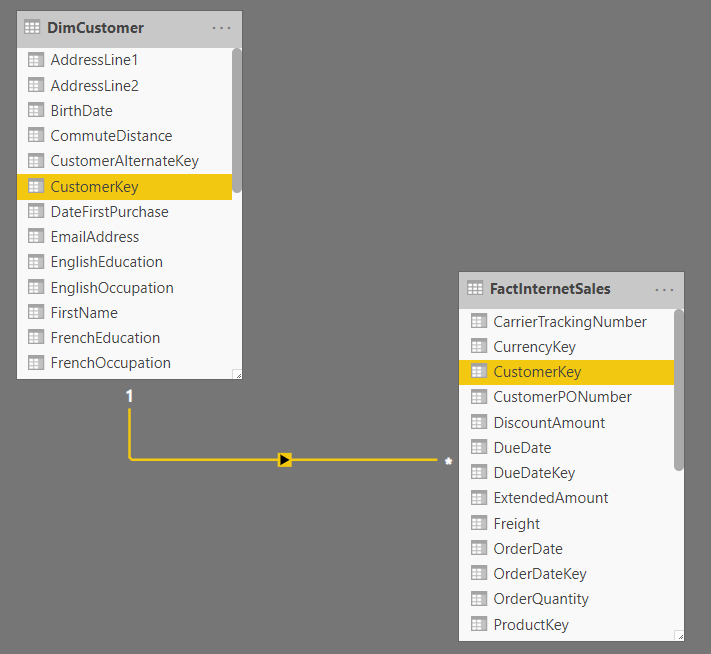The join between FactInternetSales and DimCustomer can be made using column CustomerKey.

So, the first step is to write a sql query that can fetch the desired information from the SQL Server Database. So, first we will try to query DimCustomer to see which column can be used to get the age of the customer.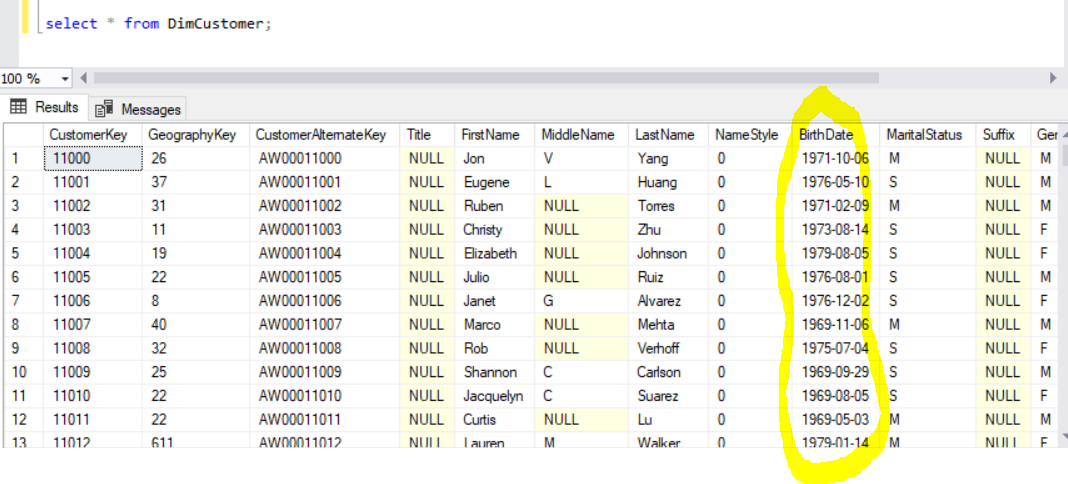From the above data we can see that the column BirthDate can be used to compute the age of the customer.

Assumption Made : The age of customer is being computed from current date. That is the difference between current date and birth date is taken as age of customer.

So, based on above analysis below is the query to get the required information :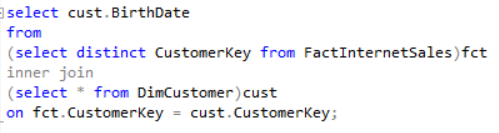Now we will write a python code to connect to AdwentureWorksDW database stored in Microsoft SQL server. To learn how to connect python to Microsoft SQL Server please refer to below blog:

https://storejockey.com/2019/04/12/how-to-connect-python-with-sql-server/

So, the python solution for the above problem would begin with making ODBC connection from python to Microsoft SQL server by using the library pyodbc. After connection is established the python code would execute the above query and fetch the results in a python data structure. The code piece for the above solution is as follows: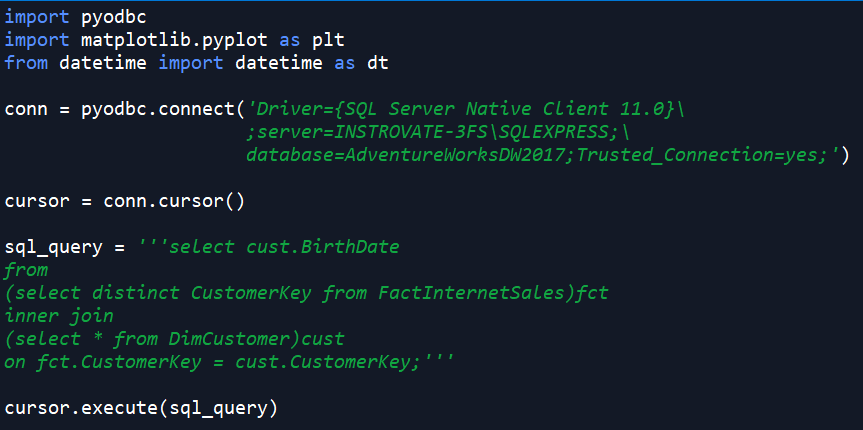The same query when executed from Microsoft SQL Server the outcome is as below:Python Data Computation Part :

We will now compute the value of age from BirthDate in Python. The piece of python code to compute the age is as follows:The list age is used to store the age of each customer. Don’t forget to import datetime library as mentioned above (In the first part of the code). Here while through each row of the query outcome, both BIrthDate and current_date are converted in to python timestamp variables. The difference between the two timestamps is stored in object diff.

The ‘days’ attribute of object ‘diff’ would the difference in terms of number of days. And the number of days divided by 365 would give us the value in years which is equivalent to the desired age of the customer.

Now the next step is to utilise the matplotlib library of python to prepare the visualization. The below is the code part to plot histogram and prepare visualization :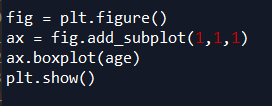Below is the visualization outcome form the above code :Box Plot Analysis :

From the above box plot we can clearly judge out targeted customers from the Box in the visualization. The customers with age group 35 and 55 are targeted customers which buys the product most. And the age group above 80 and below 30 are outliers as they buys the product least.

This analysis can be represented as below: